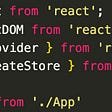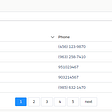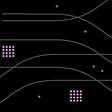# AssemblyScript Introduction (Part 1)

• The custom section (ID is 0) stores auxiliary information such as function names. Such information does not affect the execution semantics of Wasm, and there won’t be any problem even if it is completely discarded.
• The type section (ID is 1) stores function types (also called function signature) and block types.
• The import section (ID is 2) stores the import information. There are four types of items that can be imported: functions, table, memory, and global variables.
• The function section (ID is 3) stores the function signature information defined internally. This is an index table, which stores the index of the signature of the internally defined function in the type section.
• Table section (ID is 4) stores internally defined table information. Restricted by the Wasm specification, the module can import or define only one table.
• The memory section (ID is 5) stores internally defined memory information. Restricted by the Wasm specification, the module can import or define only one block of memory.
• The global section (ID is 6) stores internally defined global variable information.
• The export section (ID is 7) stores export information. Like the import section, there are four types of items that can be exported: functions, table, memory, and global variables.
• The Start section (ID is 8) stores the start function index.
• The element section (ID is 9) stores the initialization data of the table.
• The code section (ID is 10) stores the local variable information and bytecode of the internally defined functions.
• The data section (ID is 11) stores initialization data of the memory.

# Type Section

`declare function f1(x: i32): i32;declare function f2(x: f32, y: f32): f32;declare function f3(x: f32, y: f32): f32;export function f4(a: i32, b: i32): i32 {  return f1(b) + f1(b);}export function f5(a: f32, b: f32, c: f32): f32 {  return f2(a, b) + f3(b, c);}`
`(module  (type (;0;) (func (param f32 f32) (result f32)))  (type (;1;) (func (param i32) (result i32)))  (type (;2;) (func (param i32 i32) (result i32)))  (type (;3;) (func (param f32 f32 f32) (result f32)))  (import "index" "f1" (func (;0;) (type 1)))  (import "index" "f2" (func (;1;) (type 0)))  (import "index" "f3" (func (;2;) (type 0)))  (func (;3;) (type 2) (; unrelated code ;) )  (func (;4;) (type 3) (; unrelated code ;) )  ;; unrelated code)`

# Import & Export Section

`declare function add(a: i32, b: i32): i32;@external("sub2")declare function sub(a: i32, b: i32): i32;@external("math", "mul2")declare function mul(a: i32, b: i32): i32;@external("math", "pi")declare const pi: f32;export function main(): void {  add(1, 2);  sub(1, 2);  mul(1, pi as i32);}`
`(module  (type (;0;) (func (param i32 i32) (result i32)))  (type (;1;) (func))  (import "index" "add" (func (;0;) (type 0)))  (import "index" "sub2" (func (;1;) (type 0)))  (import "math" "mul2" (func (;2;) (type 0)))  (import "math" "pi" (global (;0;) f32))  (import "env" "memory" (memory (;0;) 0))  (import "env" "table" (table (;0;) 1 funcref))  (func (;3;) (type 1) (; unrelated code ;) )  ;; unrelated code)`
`export const pi: f32 = 3.14;export function add(a: i32, b: i32): i32 { return a + b; }export function sub(a: i32, b: i32): i32 { return a - b; }export function mul(a: i32, b: i32): i32 { return a * b; }`
`(module  (type (;0;) (func (param i32 i32) (result i32)))  (func (;0;) (type 0) (; unrelated code ;) )  (func (;1;) (type 0) (; unrelated code ;) )  (func (;2;) (type 0) (; unrelated code ;) )  (table (;0;) 1 funcref)  (memory (;0;) 0)  (global (;0;) f32 (f32.const 0x1.91eb86p+1 (;=3.14;)))  (export "memory" (memory 0))  (export "table" (table 0))  (export "pi" (global 0))  (export "add" (func 0))  (export "sub" (func 1))  (export "mul" (func 2))  (elem (;0;) (i32.const 1) func))`

# Function & Code Section

`function add(a: i32, b: i32): i32 { return a + b; }function sub(a: i32, b: i32): i32 { return a - b; }function mul(a: i32, b: i32): i32 { return a * b; }export function main(): void {  add(1, 2);  sub(1, 2);  mul(1, 2);}`
`(module  (type (;0;) (func (param i32 i32) (result i32)))  (type (;1;) (func))  (func (;0;) (type 0) (; unrelated code ;) )  (func (;1;) (type 0) (; unrelated code ;) )  (func (;2;) (type 0) (; unrelated code ;) )  (func (;3;) (type 1) (; unrelated code ;) )  (table (;0;) 1 funcref)  (memory (;0;) 0)  (export "memory" (memory 0))  (export "main" (func 3))  (elem (;0;) (i32.const 1) func))`
`...Section Details:Type: - type (i32, i32) -> i32 - type () -> nilFunction: - func sig=0 - func sig=0 - func sig=0 - func sig=1 <main>Table: ...Memory: ...Export: ...Elem: ...Code: - func size=7 - func size=7 - func size=7 - func size=23 <main>Custom: ...`

# Table & Element Section

`type op = (a: i32, b: i32) => i32;function add(a: i32, b: i32): i32 { return a + b; }function sub(a: i32, b: i32): i32 { return a - b; }function mul(a: i32, b: i32): i32 { return a * b; }export function calc(a: i32, b: i32, op: (x:i32, y:i32) => i32): i32 {  return op(a, b);}export function main(a: i32, b: i32): void {  calc(a, b, add);  calc(a, b, sub);  calc(a, b, mul);}`
`(module  (type (;0;) (func (param i32 i32) (result i32)))  (type (;1;) (func (param i32 i32)))  (type (;2;) (func (param i32 i32 i32) (result i32)))  (func (;0;) (type 2) (param i32 i32 i32) (result i32)    (call_indirect (type 0)      (local.get 0) (local.get 1)      (block (result i32)  ;; label = @1        (global.set 0 (i32.const 2))        (local.get 2)      )    )  )  (func (;1;) (type 0) (; unrelated code ;) )  (func (;2;) (type 0) (; unrelated code ;) )  (func (;3;) (type 0) (; unrelated code ;) )  (func (;4;) (type 1) (param i32 i32)    (drop (call 0 (local.get 0) (local.get 1) (i32.const 1)))    (drop (call 0 (local.get 0) (local.get 1) (i32.const 2)))    (drop (call 0 (local.get 0) (local.get 1) (i32.const 3)))  )  (table (;0;) 4 funcref)  (memory (;0;) 0)  (global (;0;) (mut i32) (i32.const 0))  (export "memory" (memory 0))  (export "calc" (func 0))  (export "main" (func 4))  (elem (;0;) (i32.const 1) func 1 2 3))`

# Memory & Data Section

`declare function printChar(c: i32): void;export function main(): void {  const str = "Hello, World!\n";  for (let i = 0; i < str.length; i++) {    printChar(str.charCodeAt(i));  }}`
`(module  (type (;0;) (func))  (type (;1;) (func (param i32)))  (import "index" "printChar" (func (;0;) (type 1)))  (func (;1;) (type 0) (; unrelated code ;) )  (memory (;0;) 1)  (export "memory" (memory 0))  (export "main" (func 1))  (data (;0;) (i32.const 1024) "\1c\00\00\00\01\00\00\00\01\00\00\00\1c\00\00\00H\00e\00l\00l\00o\00,\00 \00W\00o\00r\00l\00d\00!\00\0a"))`

# Global Section

`var g1: i32 = 100;export var g2: i32 = 200;export var g3: i64 = 300;export const pi: f32 = 3.14;export function main(): i32 {  return g1;}`
`(module  (type (;0;) (func (result i32)))  (func (;0;) (type 0) (result i32) (global.get 0))  (table (;0;) 1 funcref)  (memory (;0;) 0)  (global (;0;) (mut i32) (i32.const 100))  (global (;1;) (mut i32) (i32.const 200))  (global (;2;) (mut i64) (i64.const 300))  (global (;3;) f32 (f32.const 0x1.91eb86p+1 (;=3.14;)))  (export "memory" (memory 0))  (export "g2" (global 1))  (export "g3" (global 2))  (export "pi" (global 3))  (export "main" (func 0))  (elem (;0;) (i32.const 1) func))`

# Start Section

`declare function max(a: i32, b: i32): i32;declare function printI32(n: i32): void;var x = max(123, 456);export function main(): void {  printI32(x);}`
`(module  (type (;0;) (func))  (type (;1;) (func (param i32)))  (type (;2;) (func (param i32 i32) (result i32)))  (import "index" "max" (func (;0;) (type 2)))  (import "index" "printI32" (func (;1;) (type 1)))  (func (;2;) (type 0)    (global.set 0 (call 0 (i32.const 123) (i32.const 456)))  )  (func (;3;) (type 0) (call 1 (global.get 0)))  (func (;4;) (type 0) (call 2))  (table (;0;) 1 funcref)  (memory (;0;) 0)  (global (;0;) (mut i32) (i32.const 0))  (export "memory" (memory 0))  (export "main" (func 3))  (start 4)  (elem (;0;) (i32.const 1) func))`

# Custom Section

`...Section Details:Type: - type () -> nil - type (i32) -> nil - type (i32, i32) -> i32Import: - func sig=2 <assembly/index/max> <- index.max - func sig=1 <assembly/index/printI32> <- index.printI32Function: - func sig=0 <start:assembly/index> - func sig=0 <assembly/index/main> - func sig=0 <~start>Table: ...Memory: ...Global: - global i32 mutable=1 - init i32=0Export: ...Start: - start function: 4Elem: ...Code: - func size=12 <start:assembly/index> - func size=6 <assembly/index/main> - func size=4 <~start>Custom: - name: "name" - func <assembly/index/max> - func <assembly/index/printI32> - func <start:assembly/index> - func <assembly/index/main> - func <~start>Custom: - name: "sourceMappingURL"`

# Summary

--

--

--

## More from CoinEx Smart Chain

A public chain built for the decentralized exchange. Website: www.coinex.org Telegram: t.me/CoinExChain

Love podcasts or audiobooks? Learn on the go with our new app.

## Seek and Destroy — The JavaScript Algorithm## Running a Scheduled Event Using AWS Lambda, EventBridge Rules, and CDK## How much should programmers charge for their service?## Setting up Redux in your React Application## Lightning Web Component (LWC) -Pagination## How to Event Stream Data from Your Eleventy Site to Google Analytics Using RudderStack## CoinEx Smart Chain

A public chain built for the decentralized exchange. Website: www.coinex.org Telegram: t.me/CoinExChain

## COMPUTING POWER: Why Choose UncleMine## Unmarshal’s Refer and Earn Campaign## Introducing Ankr## Tokenization — Scallop Learn